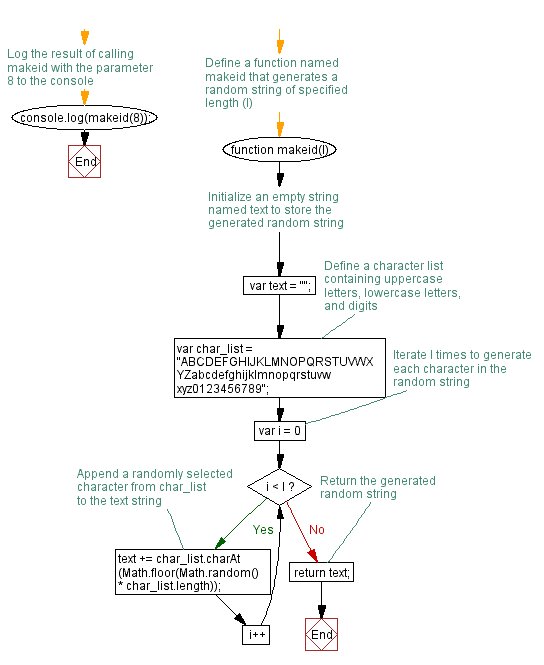# JavaScript: Generates a string id (specified length) of random characters

## JavaScript Function: Exercise-20 with Solution

Write a JavaScript function that generates a string ID (specified length) of random characters.

Sample character list : "ABCDEFGHIJKLMNOPQRSTUVWXYZabcdefghijklmnopqrstuvwxyz0123456789"

Sample Solution: -

HTML Code:

``````<!DOCTYPE html>
<html>
<meta charset=utf-8 />
<title>Generates a string id (specified length) of random characters</title>
<body>

</body>
</html>
```
```

JavaScript Code:

``````function makeid(l)
{
var text = "";
var char_list = "ABCDEFGHIJKLMNOPQRSTUVWXYZabcdefghijklmnopqrstuvwxyz0123456789";
for(var i=0; i < l; i++ )
{
text += char_list.charAt(Math.floor(Math.random() * char_list.length));
}
return text;
}
console.log(makeid(8));
```
```

Sample Output:

```4SGqCfrz
```

Flowchart:Live Demo:

See the Pen JavaScript - Generates a string id (specified length) of random characters-function-ex- 20 by w3resource (@w3resource) on CodePen.

What is the difficulty level of this exercise?

Test your Programming skills with w3resource's quiz.

﻿

## JavaScript: Tips of the Day

Import keyword

```// index.js
console.log('running index.js');
import { sum } from './sum.js';
console.log(sum(1, 2));

// sum.js
console.log('running sum.js');
export const sum = (a, b) => a + b;
```

With the import keyword, all imported modules are pre-parsed. This means that the imported modules get run first, the code in the file which imports the module gets executed after.
This is a difference between require() in CommonJS and import! With require(), you can load dependencies on demand while the code is being run. If we would have used require instead of import, running index.js, running sum.js, 3 would have been logged to the console.

Ref: https://bit.ly/323Y0P6

We are closing our Disqus commenting system for some maintenanace issues. You may write to us at reach[at]yahoo[dot]com or visit us at Facebook